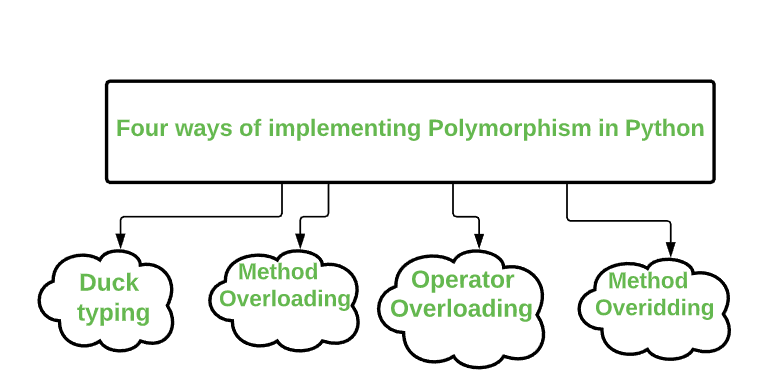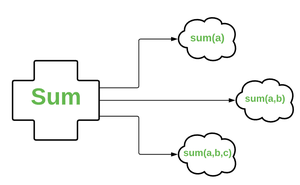# Ways of implementing Polymorphism in Python

• Difficulty Level : Expert
• Last Updated : 30 Jun, 2021

In programming, Polymorphism is a concept of Object-Oriented Programming. It enables using a single interface with the input of different data types, different classes or maybe for a different number of inputs.

Example: In this case, the function len() is polymorphic as it is taking a string as input in the first case and is taking a list as input in the second case.

Attention geek! Strengthen your foundations with the Python Programming Foundation Course and learn the basics.

To begin with, your interview preparations Enhance your Data Structures concepts with the Python DS Course. And to begin with your Machine Learning Journey, join the Machine Learning - Basic Level Course

## Python3

 `# length of string``x ``=` `len``(``'Geeks'``)``print``(x)` `# length of list``y ``=` `len``([``1``, ``2``, ``3``, ``4``])``print``(y)`

Output:

```5
4```

In Python, Polymorphism is a way of making a function accept objects of different classes if they behave similarly.

There are four ways of implementing Polymorphism in Python:1. Duck Typing: Duck typing is a concept that says that the “type” of the object is a matter of concern only at runtime and you don’t need to explicitly mention the type of the object before you perform any kind of operation on that object, unlike normal typing where the suitability of an object is determined by its type.

In Python, we have the concept of Dynamic typing i.e we can mention the type of variable/object later. The idea is that you don’t need a type in order to invoke an existing method on an object if a method is defined on it, you can invoke it.

## Python3

 `class` `Geeks1:``  ``def` `code (``self``, ide):``    ``ide.execute()``    ` `class` `Geeks2:``  ``def` `execute (``self``):``    ``print``(``"GeeksForGeeks is the best Platform for learning"``)``    ` `# create object of Geeks2   ``ide ``=` `Geeks2()` `# create object of class Geeks1``G1 ``=` `Geeks1()` `# calling the function by giving ide as the argument.``G1.code(ide)`

Output:

`GeeksForGeeks is the best Platform for learning`

Given a single function sum (), the number of parameters can be specified by you. This process of calling the same method in different ways is called method overloading.## Python3

 `class` `GFG:``    ``def` `sum``(``self``, a ``=` `None``, b ``=` `None``, c ``=` `None``):        ``        ``s ``=` `0``        ``if` `a !``=` `None` `and` `b !``=` `None` `and` `c !``=` `None``:``            ``s ``=` `a ``+` `b ``+` `c``        ``elif` `a !``=` `None` `and` `b !``=` `None``:``            ``s ``=` `a ``+` `b``        ``else``:``            ``s ``=` `a``        ``return` `s``      ` `s ``=` `GFG()` `# sum of 1 integer``print``(s.``sum``(``1``))` `# sum of 2 integers``print``(s.``sum``(``3``, ``5``))` `# sum of 3 integers``print``(s.``sum``(``1``, ``2``, ``3``))`

Output:

```1
8
6```

3. Operator Overloading: Operator overloading in Python is the ability of a single operator to perform more than one operation based on the class (type) of operands. So, basically defining methods for operators is known as operator overloading. For e.g: To use the + operator with custom objects  you need to define a method called __add__.

We know + operator is used for adding numbers and at the same time to concatenate strings. It is possible because the + operator is overloaded by both int class and str class. The operators are actually methods defined in respective classes.

So if you want to use the + operator to add two objects of some user-defined class then you will have to define that behavior yourself and inform Python about that.

## Python3

 `class` `Student:``    ``def` `__init__(``self``, m1, m2):``        ``self``.m1 ``=` `m1``        ``self``.m2 ``=` `m2` `S1 ``=` `Student (``58``, ``60``)``S2 ``=` `Student (``60``, ``58``)` `# this will generate an error``S3 ``=` `S1 ``+` `S2`

Output:

`TypeError: unsupported operand type(s) for +: 'Student' and 'Student'`

So we can see that the + operator is not supported in a user-defined class. But we can do the same by overloading the + operator for our class.

## Python3

 `class` `Student:``  ` `    ``# defining init method for class``    ``def` `__init__(``self``, m1, m2):``        ``self``.m1 ``=` `m1``        ``self``.m2 ``=` `m2` `    ``# overloading the + operator``    ``def` `__add__(``self``, other):``        ``m1 ``=` `self``.m1 ``+` `other.m1``        ``m2 ``=` `self``.m2 ``+` `other.m2``        ``s3 ``=` `Student(m1, m2)``        ``return` `s3` `s1 ``=` `Student(``58``, ``59``)``s2 ``=` `Student(``60``, ``65``)``s3 ``=` `s1 ``+` `s2``print``(s3.m1)`

Output:

`118`

4. Method Overriding: By using method overriding a class may “copy” another class, avoiding duplicated code, and at the same time enhance or customize a part of it. Method overriding is thus a part of the inheritance mechanism.

In Python, method overriding occurs by simply defining the child class in a method with the same name as a method in the parent class.

## Python3

 `# parent class``class` `Programming:``  ` `    ``# properties``    ``DataStructures ``=` `True``    ``Algorithms ``=` `True``    ` `    ``# function practice``    ``def` `practice(``self``):``        ``print``(``"Practice makes a man perfect"``)``    ` `    ``# function consistency``    ``def` `consistency(``self``):``        ``print``(``"Hard work along with consistency can defeat Talent"``)``        ` `# child class       ``class` `Python(Programming):``    ` `    ``# function``    ``def` `consistency(``self``):``        ``print``(``"Hard work along with consistency can defeat Talent."``)` `Py ``=` `Python()``Py.consistency()``Py.practice()`

Output:

```Hard work along with consistency can defeat Talent.
Practice makes a man perfect```

My Personal Notes arrow_drop_up